Bound State Solutions of the Klein-Gordon Equation for the More General Exponential Screened Coulomb Potential Plus Yukawa (MGESCY) Potential Using Nikiforov-Uvarov Method

# Bound State Solutions of the Klein-Gordon Equation for the More General Exponential Screened Coulomb Potential Plus Yukawa (MGESCY) Potential Using Nikiforov-Uvarov Method

Louis Hitler1,2*, Ita Benedict Iserom1, Paul Tchoua3 and Akpama Arikpo Ettah1
1Physical/Theoretical Chemistry Research Group, Department of Pure and Applied Chemistry, University of Calabar, Calabar, CRS, Nigeria
2CAS Key Laboratory for Nanosystem and Hierarchical Fabrication, CAS Centre for Excellence in Nanoscience, National Centre For Nanoscience and Technology, University of Chinese Academy of Sciences, Beijing, China
3Department of Mathematics, Ngaoundere University, Ngaoundere, Cameroon
*Corresponding Author: Louis Hitler, Researcher, University of Chinese Academy of Sciences, Beijing, China, Tel: +86150-0107-5832, Email: [email protected]

Received Date: Dec 12, 2017 / Accepted Date: Feb 07, 2018 / Published Date: Feb 12, 2018

### Abstract

There has been a growing interest in investigating the approximate solutions of the Klein-Gordon equation and relativistic wave equations for some physical potential models. This is due to the fact that the analytical solutions contain all the necessary information for the quantum system under consideration. In this paper, we obtained the solutions of the Klein-Gordon equation with more general exponential screened coulomb (MGESC), Yukawa potential (YP) and the sum of the mixed potential (MGESCY) using the Parametric Nikiforov-Uvarov Method (PNUM). The bound state energy eigenvalues and the corresponding un-normalized eigenfunctions expressed in terms of hypergeometric functions are obtained.

Keywords: Yukawa; Exponential screened coulomb; Bound state; Klein-Gordon

#### Introduction

The more general exponential screened coulomb (MGESC) potential expressed as: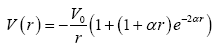(1)

is a potential of great interest which on expansion comprises of the sum of coulomb potential, modified screened coulomb or the Yukawa potential and a modified exponential potential given as: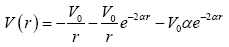(2)

This potential is known to describe adequately the effective potential of a many-body system of a variety of fields such as the atomic, solid state, plasma and quantum field theory . The problems arising from screened coulomb potential is of indubitable importance in physics and chemistry of atomic incidence. To tackle this problem, various methods have been applied both numerical and analytical. These methods include the WKB method  and various types of perturbation method [3-5]. Upon expansion of the MGESC Potential in eqn. (1) given as: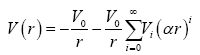(3)

The coefficient Vi of eqn. (3) can be obtained so that the perturbation method  may be applied. Recently, a novel perturbative formalism which is based on decomposing the radial part of the Schrodinger equation into two, having an Exact solvable part and approximate treatment depending on the nature of the perturbed potential  have been applied on the MGESC potential and bound state energies as well as wave functions to both bound and continuum region have been obtained. Hence, the Schrodinger equation for the MGESC Potential doesn’t admit exact solution. For this reason, Sever and Tezcan  applied the large-N expansion following the method proposed by Mlodinow and Papanicolaou  to obtain the energy eigenvalues for the ground state and the first excited state as well as their corresponding wavefuntions. Roy in 2013 carried out extensive studies on some exponential screened coulomb potentials such as the Exponential Cosine Screened Coulomb (ECSC) and General Exponential Screened Coulomb (GESC) potential with special emphasis on higher states and stronger interactions . In his speculative studies, he obtained bound state solutions of both screened potentials via the Generalized Pseudospectral (GPS) Method and computed reasonable results for the energy eigenvalues at different states compared with other results obtained in the literature. Ita and Ekuri, carried out studies on the MGESC potential for diatomic molecules to obtain boundstate solutions of the Schrodinger equation using the Nikiforov-Uvarov (NU) Method .

The Yukawa potential, in atomics and particle physics expressed in the form: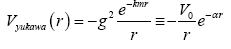(4)

where g is the magnitude scaling constant, m is the mass of the affected particle, r is the radial distance to the particles, k is another scaling constant was first proposed by Hideki Yukawa in 1935 on the paper titled “On the interaction of Elementary Particles” In his work, he explained the effect of heavy nuclei interaction on peons. According to Yukawa, he expanded that the interactions of particles is not always accompanied by emission of light particles when heavy particles are transmitted from neutron state to proton state, but the liberated energy due to the transmission is taken up sometimes by another heavy particles, which will be transformed from proton state into neutron state . The Yukawa potential is a potential that decreases more rapidly with distance and can be expressed as the coulomb potential when m→0. Since then, numerous researches had been conducted by various scientists to obtain bound state of the potential by applying different scientific Methods. Gerry and Lamb in studied the screened coulomb potential of the Yukawa type by using a scaling Variational method based on the SO(2,1) Subgroup of the full SO(4.2) dynamic group of the point coulombic problem to obtain both energy eigenvalues for different states and Normalized wave functions . Gerry and Lamb, applied the large-N phase Integral approximation based on the coherent states of SO(2,1) (SU(1,1)) to coulomb-like problems where they obtained energy eigenvalue for s-states of the Yukawa potential. Hamzavi and co-workers in studied the Yukawa potential via a two body semi-relativistic (Spinless Salpeter) SS equation and obtained bound state energy values and their corresponding Normalized wavefunctions for short range Yukawa potential with arbitrary l-state using parametric NU Method. In their literature, it was spelt out that the known Static Screened Coulomb Potential (SSCP) yield reasonable results only for the innermost state when Z is Large whereas, gives rather poor result for the outermost and middle atomic states . Dutt et al. carried out studies on a screened coulomb potential by using a Rayleigh-Schrodinger Perturbation theory and obtained energy eigen values for large values of screening parameters . Their calculations to the energy eigenvalue yield reasonable result compared to other numerical and analytical methods. Hamzavi and his colleagues applied the NU Method to the Yukawa potential for any l-state and obtained bound state approximate analytical solutions . Computed values for the bound state energy for different states were obtained and compared with the (Asymptotic Iteration Method) AIM, Supersymmetric (SUSY) and Numerical method appearing in their literature. Gerry and Lamb, obtained the energy eigenvalues for the Yukawa potential by using the Generalized Scaling Variational method for a system with a spherically symmetric potential coulumbic at the origin . The energy eigenvalues for different states and different screening parameters for bound states were obtained and these values were in agreement with those obtained in the literature Dutt and Varshni, studied the energy levels of neutral atoms by applying the shifted large-N expansion to the Yukawa potential with a modified screening parameter . They obtained energy values for the k-shell over the range of atomic number Z up to 84 and compared with those obtained within the framework of hyper-viral-pad scheme and observed that the large-N techniques may also be applied in other areas of atomic physics. Sharma et al. calculated bound state for all angular momenta for superposed two static screened coulomb potentials (SSCP) expressed as :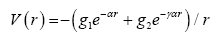(5)

Where g1 and g2 are coupling constants, α is the screening parameter and γ is the screening strength. By subjecting g1=0 the modified screened coulomb potential as well as its numerical calculations for the bound state is obtained. Pakdel et al. studied the Dirac equation with scalar and vector potential for the Yukawa potential and obtained both bound and scattering states . In their calculations, the energy eigenvalues for different values of n and k was reported numerically as well as their corresponding eigenstates. Since the screened coulomb potential plays significant role in microscopic fields, this potential have been applied in different branches of atomic and molecular physics and chemistry. For this reason, Roy, carried out studies on the critical parameters and spherical confinement of H atom in screened Coulomb potential using the GPS method . He extended his studies towards finding bound state energy eigenvalues for the screened coulomb potential: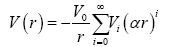(6)

and their corresponding wavefunctions as well as providing information regarding sample dipole polarizability. Onate and Ojunubah, applied the supersymmetric shape invariance approach and formalism on a class of Yukawa potential is expressed :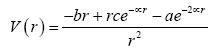(7)

and obtained bound state energy eigenvalue calculations. They deduced three different energy representations for the following potentials such as the coulomb, Yukawa and inversely quadratic Yukawa as they obtained their normalized wavefunctions and energy eigenvalues, compared to other related work via the NU and AP method in their literature and the values obtained yielded reasonable result. Ita et al., obtained bound state solutions of the Schrödinger’s equation for Manning-Rosen plus a Class of Yukawa (MRCY) potential given as :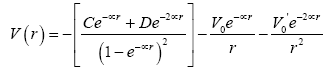(8)

From their calculations, they deduced three different potential such as the Manning-Rosen, Yukawa and inversely quadratic Yukawa potential and obtained bound state energy eigenvalues as well as wave functions for different principal quantum number n for the s-state. In the view of relativistic quantum mechanics, a particle moving in a potential field is described particularly with the Klein-Gordon (KG) equation. Over the years numerous works have been reported concerning the Klein-Gordon equations for various kinds of potentials by using different Methods such as supersymmetry, supersymmetric WKB approach, Nikiforov-Uvarov Method [21-30]. Ikhdair, obtained approximate analytical bound state solution of the Klein-Gordon equation with equal Scalar and Vector Eckart type potential given in eqn. (9) .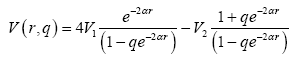(9)

via the NU Method. Both energy equation as well as the un-normalized wave functions expressed in terms of the Jacobi polynomial was obtained. Ikot et al. obtained approximate analytic solutions of the Klein-Gordon in D-dimension for any l-state for a seven parameter type potential expressed as :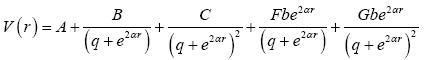(10)

where A,B,C,F and G are potential parameters, q is the deformation parameter, b=e2αre, re is the distance from equilibrium position and α is the screening parameter. In their work, they obtained both bound and scattering state with energy spectrum of some special potential such as Hulthen, Manning-Rosen, Eckart and Wood-Saxon potential. Hansabadi studied a special kratzer-type potential where:

V(r)1 S(r) and obtained bound state solution of the Klein-Gordon equation with position dependent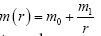as well as the wavefunction . Since then many literatures have reported different special case of potential such as, Poschl-Teller potential Rosen-Morse and many more by applying different methods. Moreover when arbitrary angular momentum quantum number l is present, one can only solve the SE and KGE approximately using suitable approximation scheme. Some of such approximations include convectional approximation scheme proposed by Greene and Aldrich improved approximation scheme elegant approximation scheme. These approximations are used to deal with the centrifugal term or potential barrier arising from the problem of interest [16,34-39]. Not much has been achieved on the Schrodinger equation for the MGESCY potential over the years. The aim of this report is to obtain bound state solutions of both Schrödinger equation for the More General Exponential Screened Coulomb Potential plus Yukawa (MGESCY) potential.

#### Theoretical Approach

The Nikiforov-Uvarov method is based on the solutions to a second-order linear differential equation with special orthogonal function . The hyper-geometric type has been used to solve the Schrödinger, klein-Gordon and Dirac equation for different kind of potentials [25-30].

The more generalized form of nikiforov-uvarov method

Given a second order differential equation of the form: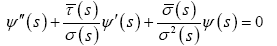(11)

In order to find the exact solutions to eqn. (11), we set the wavefunction as: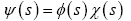(12)

And on substituting eqn. (12) into eqn. (11), then eqn. (11) reduces to hypergeometric type: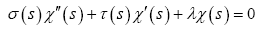(13)

where the wave function ϕ(s) is defined as the logarithmic derivative,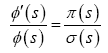(14)

where π(s) is at most first order polynomials.

Likewise, the hyper geometric type function ϕ(s) in eqn. (13) for a fixed n is given by the Rodriques relation as: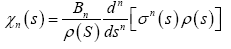(15)

where Bn is the normalization constant and the weight function ρ(s) must satisfy the condition: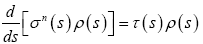(16)

with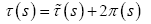(17)

In order to accomplish the condition imposed on the weight function ρ(s), it is necessary that the classical or polynomials τ(s) be equal to zero to some point of an interval (a,b) and its derivative at this interval at σ(s) > 0 will be negative, that is: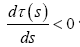(18)

Therefore, the function π(s) and the parameters λ required for the NU method are defined as follows: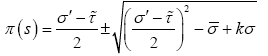(19)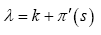(20)

The s-values in eqn. (19) are possible to evaluate if the expression under the square root be square of polynomials. This is possible, if and only if its discriminant is zero. With this, the new eigenvalues equation becomes: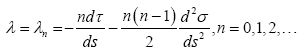(21)

On comparing eqns. (20) and (21), we can obtain the energy eigenvalues.

Parametric nikiforov-uvarov method

The parametric form is simply using parameters to obtain explicitly energy eigenvalues and it is still based on the solutions of a generalized second order linear differential equation with special orthogonal functions. The hypergeometric NU method has shown its power in calculating the exact energy levels of all bound states for some solvable quantum systems.

Given a second order differential equation of the form: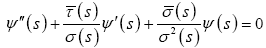(22)

Where σ(s) and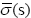are polynomials at most second degree and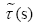is first degree polynomials. The parametric generalization of the N-U method is given by the generalized hypergeometric-type equation: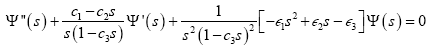(23)

Thus eqn. (22) can be solved by comparing it with eqn. (23) and the following polynomials are obtained: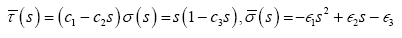(24)

The parameters obtainable from equation (23) serve as important tools to finding the energy eigenvalue and eigenfunctions.

Now substituting eqn. (24) into eqn. (19):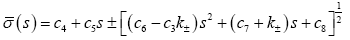(25)

where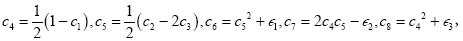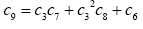(26)

The resulting value of k in eqn. (25) is obtained from the condition that the function under the square root be square of a polynomials and it yields,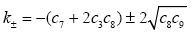(27)

The new π(s) for k_ becomes: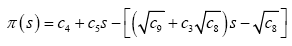(28)

for the k_ value,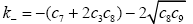(29)

Using eqn. (17), we obtain: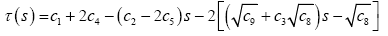(30)

The physical condition for the bound state solution is τ'< 0 and thus: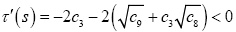(31)

with the aid of eqns. (20) and (21), we obtain the energy equation as: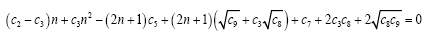(32)

The weight function ρ(s) is obtained from eqn. (16) as: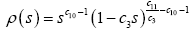(33)

and together with eqn. (15), we have: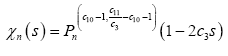(34)

where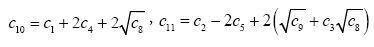(35)

and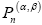are the Jacobi polynomials. The second part of the wave function is obtained from eqn. (14) as: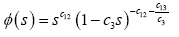(36)

where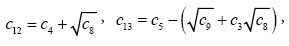(37)

Thus, the total wave function becomes: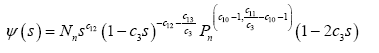(38)

where Nn is the normalization constant.

#### The Klein-Gordon Equation

Given the Klein-Gordon equation as [25-27]: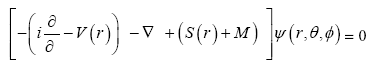(39)

If one assigns the corresponding spherical total wave function: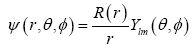(40)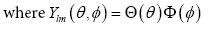(41)

then the wave equation is separated into variables and the following equations are obtained: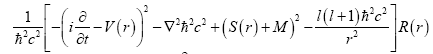(42)

where M is the rest mass,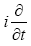is energy eigen value, V(r) and S(r)are the vector and scalar potentials respectively: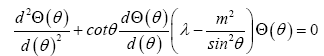(43)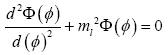(44)

Where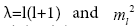are the separation constants and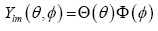is the solution of eqn. (43) and eqn. (44)

For the NU method to be applicable, eqn. (42) should be transformed into eqn. (22) to be solvable.

Solution of the radial part of the klein-gordon equation with more general exponential screened coulomb potential

Given the Klein-gordon equation as: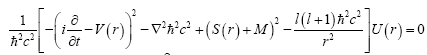(45)

where M is the rest mass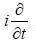is energy eigen value V(r) and S(r) are the vector and scalar potentials respectively.

The radial part of the Klein-Gordon Equation with vector V(r) potential=scalar S(r) potential in atomic units (ħ=c=1) is given as: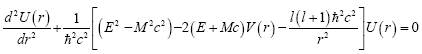(46)

eqn. (46) should be transformed into eqn. (22) to be solvable. Hence, using the transformation U(r)=rR(r), we obtain: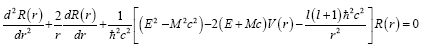(47)

Given the More General Exponential Screened Coulomb (MGESC) potential as: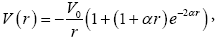(48)

where V0 is the potential depth of the MGESC potential and α is an adjustable positive parameter.

The MGESC potentials can also be expressed as: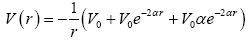(49)

Including the centrifugal term, the effective potential Veff (r) of the MGESC potential can be expressed as: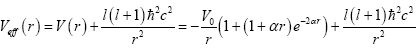(50)

Substituting potential of eqn. (49) into radial Klein-Gordon equation of eqn. (47), we obtain: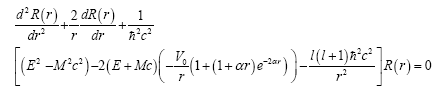(51)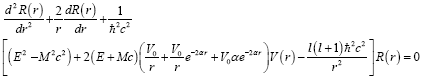(52)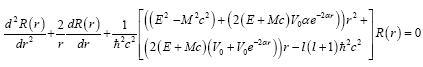(53)

Introducing the following dimensional parameters: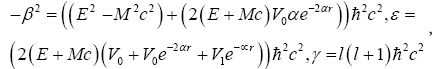(54)

Then eqn. (53) can be given as: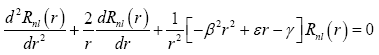(55)

eqn. (55), is a simplified form of the second order differential equation in eqn. (23). Comparing eqn. (55) and solving explicitly, we obtain the Energy eigenvalues of the KGE for the MGESC potential as: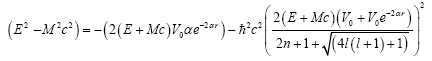(56)

and the Radial wavefunction of the KGE for the MGESC potential as: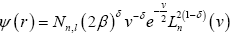(57)

Where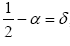n is a positive integer and Nn,l is the normalization constant:

Solutions to the radial part of the klein-gordon equation for the yukawa potential

The radial part of the Klein-Gordon Equation with vector V(r) potential=scalar S(r) potential in atomic units (ħ=c=1) is given as: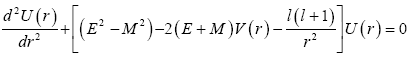(58)

Given the Yukawa potential (YP) as: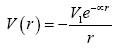(59)

Substituting potential of eqn. (59) into the Klein-Gordon equation of eqn. (58), we obtain: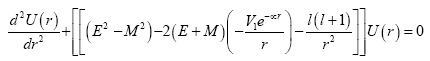(60)

Since the Klein-Gordon equation with above combine potentials rarely has exact analytical solution, an approximation to the centrifugal term has to be made. The good approximation for 1/r2 in the centrifugal barrier is taken as: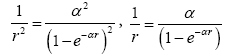(61)

Similar to other related work

Making the transformation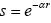eqn. (59) becomes: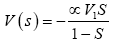(62)

Again, applying the transformation s=e-αr to get the form that NU method is applicable, eqn. (60) gives a generalized hypergeometrictype equation as: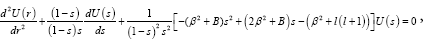(63)

Where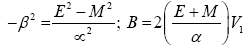(64)

Comparing eqn. (63) and solving explicitly, we obtain the energy eigenvalues of the KGE for the Yukawa potential as: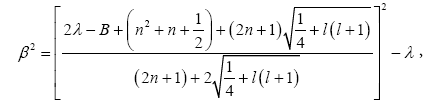(65)

eqn. (65) can be solved explicitly and the energy eigen spectrum of YP becomes: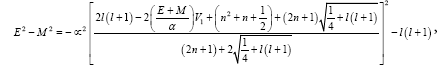(66)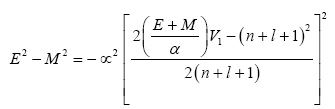(67)

Hence, the wavefunction is given bellow as: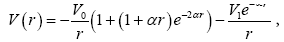(68)

where Nn,l is the normalization constant:

Solutions to the radial part of the klein-gordon equation for the more general exponential screened coulomb plus yukawa (mgescy) potential

By combining eqns. (1) and (4), we obtained an expression for mixed potentials given as: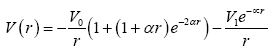(69)

Substitutin eqn. (69) into eqn. (47) gives: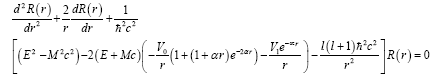(70)

we obtained both bound state solution as well as un-normalized wave function of the Klein-Gordon equation after solving eqn. (70) explicitly by applying the NU method as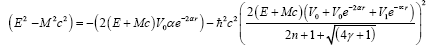(71)

and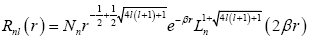(72)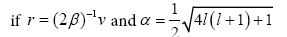(73)

substituting eqn. (73) into eqn. (72), we obtain: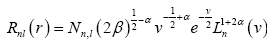(74)

where Nn,l is the normalization constant.

#### Conclusion

The analytical solutions of Klein-Gordon equation for the more general exponential screened coulomb plus yukawa (MGESCY) potential has been presented via the NU method. The Nikiforov- Uvarov (NU) method employed in the solutions enables us to explore an effective way of obtaining the energy eigenvalues and their corresponding eigenfunctions in terms of Jacobi polynomials.

#### References

Citation: Hitler L, Iserom IB, Tchoua P, Ettah AA (2018) Bound State Solutions of the Klein-Gordon Equation for the More General Exponential Screened Coulomb Potential Plus Yukawa (MGESCY) Potential Using Nikiforov-Uvarov Method. J Phys Math 9: 261. DOI: 10.4172/2090-0902.1000261

Copyright: © 2018 Hitler L, et al. This is an open-access article distributed under the terms of the Creative Commons Attribution License, which permits unrestricted use, distribution, and reproduction in any medium, provided the original author and source are credited.

Select your language of interest to view the total content in your interested language

### 2nd International Conference On Astronomy, Astrophysics & Astrobiology

Auckland, New Zealand
##### Recommended Journals
Viewmore
###### Article Usage
• Total views: 1873
• [From(publication date): 0-2018 - Feb 24, 2020]
• Breakdown by view type
• HTML page views: 1759Can't read the image? click here to refresh